Reshaping files from the World Development Indicators and datasets with similar format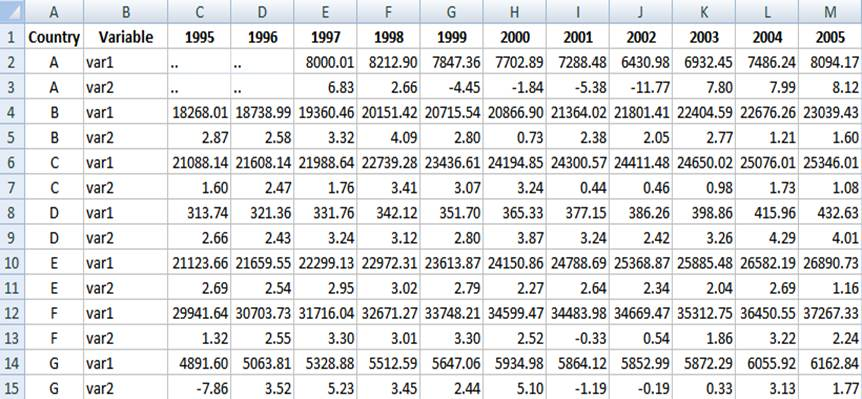Before putting this data in Stata you need to do some editing. First, you need to add a character to the column headings. Stata does not take numbers as variable names. In this case we add an “x” to the years. In excel you do this by using the ‘replace’ function. For the 1900s we replace “19” for “x19”, same for the 2000s. See the following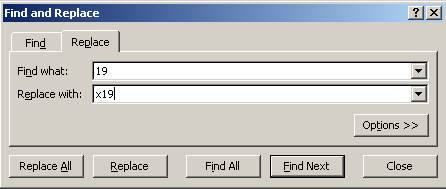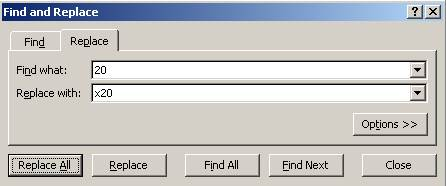So we have something like this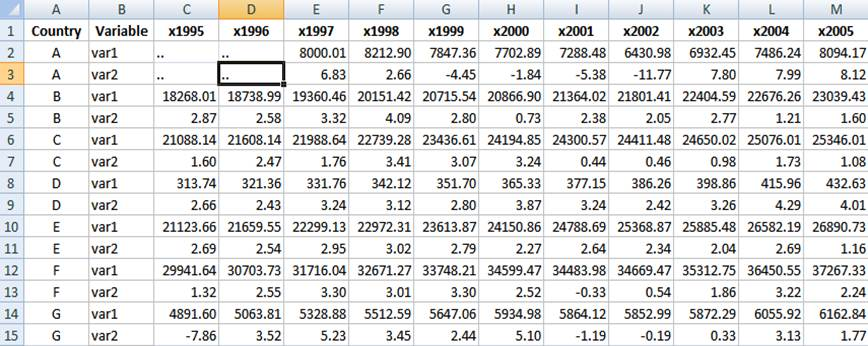Replace the dots “..” (or any string character) with a blank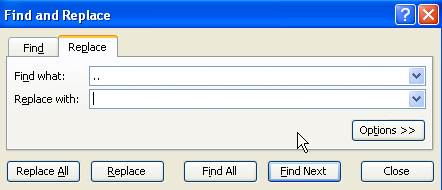Make sure the numbers are numbers. Go to Format  - Cells and select “Number” in the “Number” tab and click OK. This will format the data as numeric with two decimals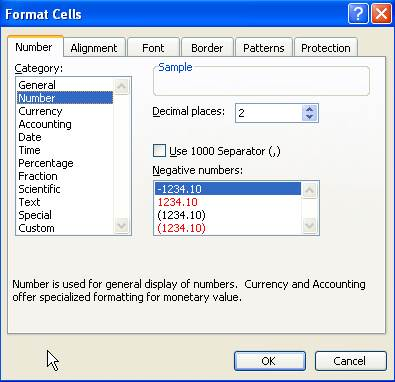The table should look like: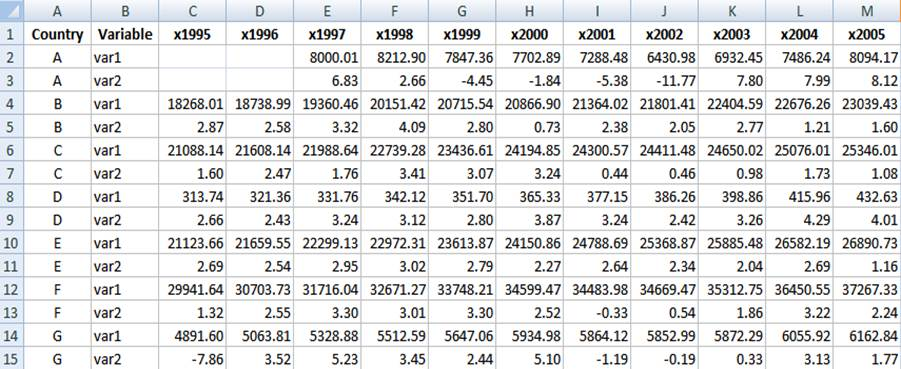Now select the whole data set, press Ctrl-C, go to the Stata’s data  editor and press Ctrl-V to paste the data, you should have the following: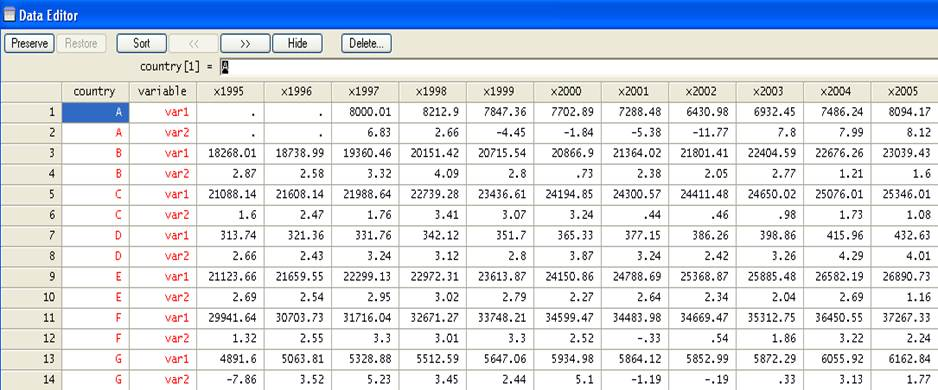Another alternative is to save the file as *.csv format. We will save here as GDPTest.csv

Open Stata and type in the command window

insheet using gdptest.csv

The Stata screen should look like this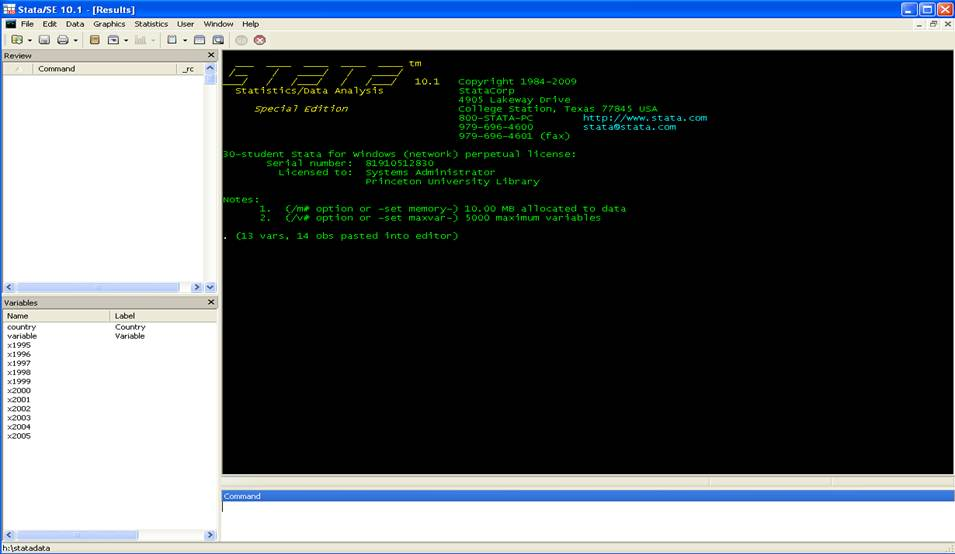Reshaping wide to long

In wide to long from

 id x2001 x2002 x2003 1 2 7 1 2 3 5 9 3 1 1 8

To this you type:

reshape long x, i(id) j(year)

 id year x 1 2001 2 1 2002 7 1 2003 1 2 2001 3 2 2002 5 2 2003 9 3 2001 1 3 2002 1 3 2003 8

First create a unique identifier for each row of data. Type:

gen id = _n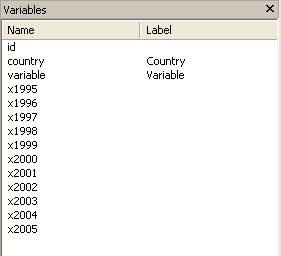Years are organized by columns and variables as observations which are not suitable for time series analysis or other type of analysis. We need to put it in a way where each year represents an observation (row) and each column has a variable. Type:

reshape long x, i(id) j(year)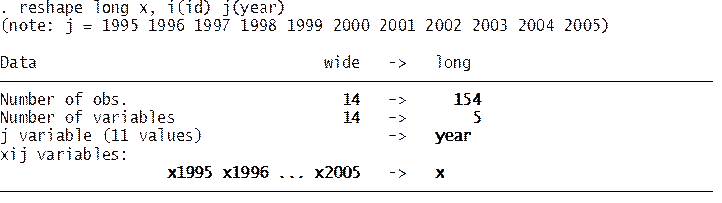Where:

• longGoes from wide to long format.
• x – The variables with the prefix “x” (x1960, x1961, x1962, etc.) are to be converted from wide to long.
• i(id) – A unique identifier for the wide format is in variable “id”.
• j(year) – Indicates that the suffix of “x” (x1961, x1962, x1963, …), the years, should be put in variable called “year”.

NOTE: If you have more than one variable you can list them as follows:

reshape long x y z, i(id) j(year)

If you browse your data, it should look like this, variable “x” has all the values for both var1 and var2.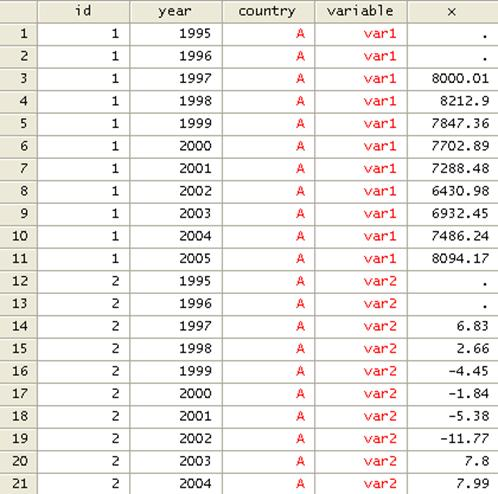To separate var1 and var2 we need to do a little bit of work.

First we need to create a new variable with the labels of each variable, type

encode variable, gen(varlabel)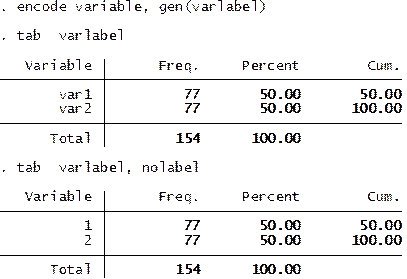Create a do-file with the labels for each variable. This comes in handy when dealing with a lot of variables.

label save varlabel using varname, replace

You will notice that varname.do is created.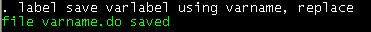Using notepad or the do-file editor within Stata open varname.do. Using the do-file editor this is what you will see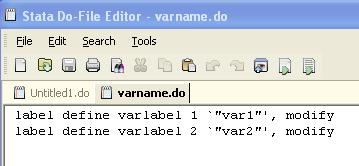Do the following changes:

1.    Change “label define” to “label variable”

2.    Change “varlabel 1” to “x1” and “varlabel 2” to “x2”

3.    Delete “, modify”

4.    Save the do-file.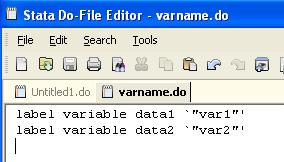Separating two stacked variables

You need to create an id variable to identify the groups. The combination of egen and group creates an id variable for the two time series within each group (‘var1’ and ‘var2’). Type

egen id2 = group(country year)

move id2 year

drop id

drop variable

To reshape long to wide you should have a table that looks like the following where one variable holds two different variables per country. Each country has two variables from 1960 to 2006.

Now let’s reshape from long to wide because we want our variables to have one column each. Type

reshape wide x, i(id2) j(varlabel)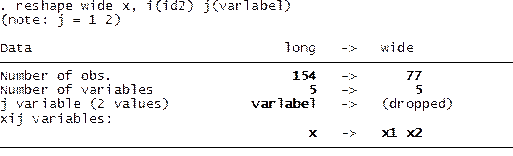Where:

• wideGoes from long to wide format.
• x   The variable of interest to go from long to wide is called “data”.
• i(id) – A unique identifier for the wide format is in variable “id”.
• j(varlabel) – Indicates that the suffix of “data” has to be taken from “”varlabel” (“varlabel” has two categories: 1 –var1- and 2 –var2).

NOTE: If “j” is not available in your dataset, you may be able to generate one using the following command:

bysort id: gen jvar=_n

Then reshape

reshape wide data, i(id) j(jvar)

Run the do-file varname.do by selecting all and clicking on the last icon, this will change the labels for x1 and x2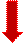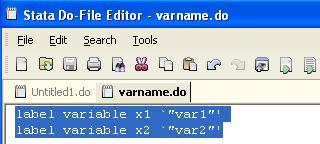The variable window should look like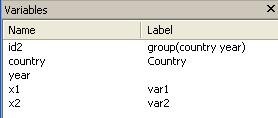The final dataset should look like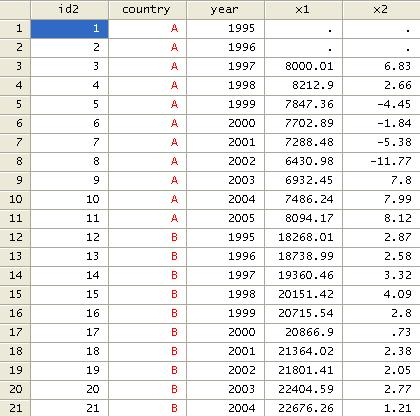Reshaping long to wide

In long to wide we go from

 id time r 1 1 2 1 2 7 1 3 1 2 1 3 2 2 5 2 3 9 3 1 1 3 2 1 3 3 8

To this

 id r.time1 r.time2 r.time3 1 2 7 1 2 3 5 9 3 1 1 8

For the example above you tipe:

reshape wide r, i(id) j(time)

Here is a more complex example.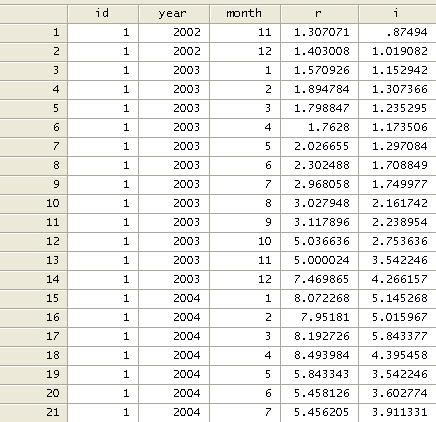Where “r” is return rate and “i” is interest rate.

IN THIS EXAMPLE WE NEED TO WORK ON THE DATE VARIABLE FIRST. IF YOU DO NOT HAVE THIS YOU CAN SKIP THIS PART.

Notice that time is separated in years and months (monthly data). We need to combine them into one date variable. Since they are numeric variables we need to put them together as string variables. We convert them to string first and then we concatenate them. Type

tostring month year, replace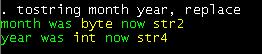We then concatenate them. Type

gen date=year+"_0"+month if length(month)==1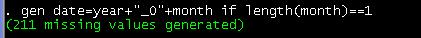This will create the date variable for dates with months 1 to 9 (with “0” before to make it double digit), see below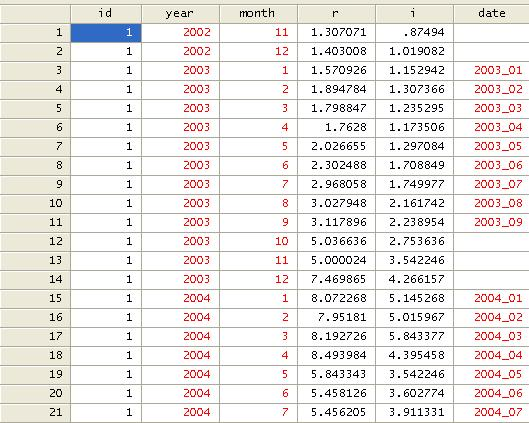Now we add the rest. Type

replace date=year+"_"+month if date==""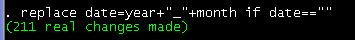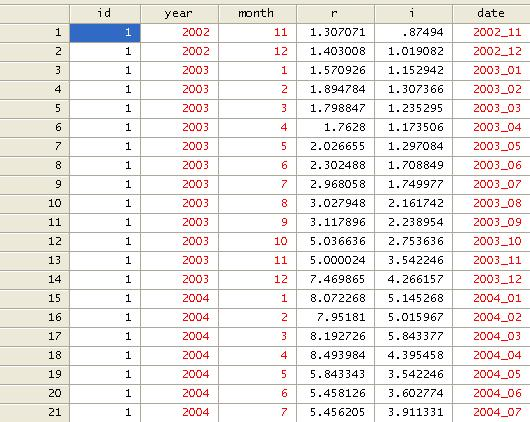We can drop ‘year’ and ‘month’.

drop year month

Let’s put some order, type

order id date

RESHAPING WIDE TO LONG

Now, let’s reshape. Type

reshape wide r i, i(id) j(date) str

We add ‘str’ because ‘date’ is a string variable.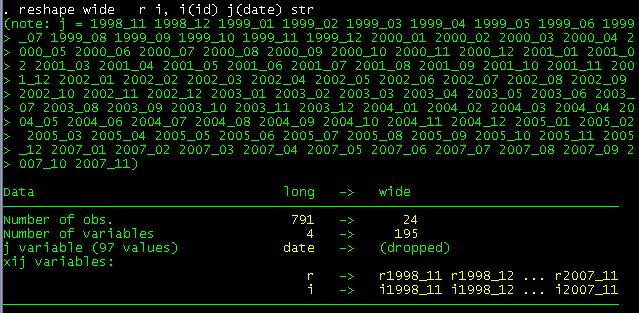Where:

• wideIndicates the type of reshape, in this case from long to wide format.
• r i    The variables of interest from long to wide are “r” and “i” (prefix for the new variables).
• i(id) – A unique identifier for the wide format is in variable “id”.
• j(date) – Indicates the suffix of “r” and “i” taken from ”date” (notice “xij” variables:” above)

The variable window should look like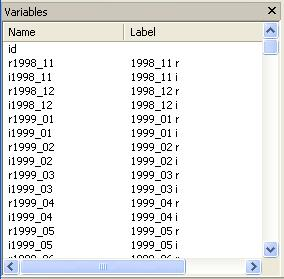And the data is now in the following format: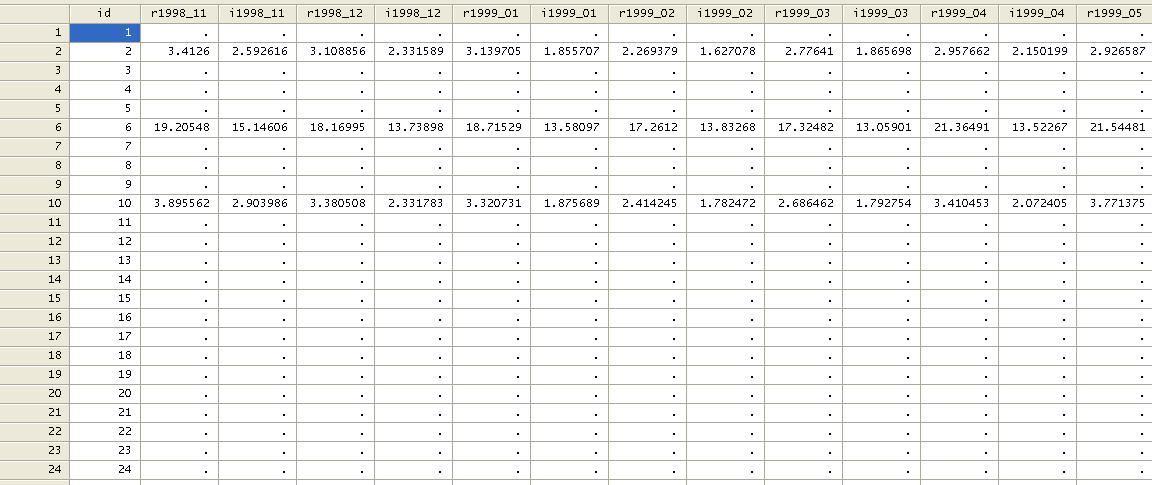NOTICE ABOVE ‘RETURNS’ AND ‘INTEREST RATES’ ARE TOGETHER FOR THE SAME TIME PERIOD. IF YOU WANT TO HAVE ALL ‘RETURNS’ AND ‘INTEREST RATES’ TOGETHER YOU NEED TO DO THE FOLLOWING:

We need to transpose the data as follows

xpose, clear varname

The data will be transposed with an additional variable “_varname” (option varname after comma)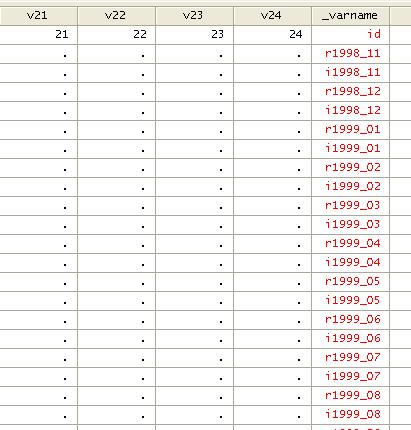We then sort by _varname

sort _varname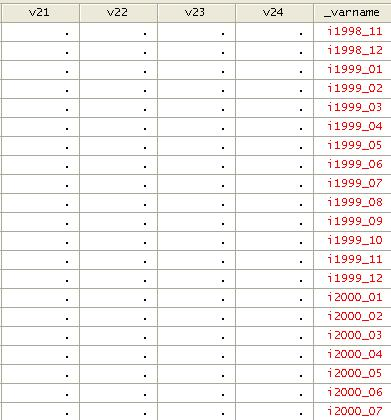And transpose again as follows:

xpose, clear

Move “id” to the top

order id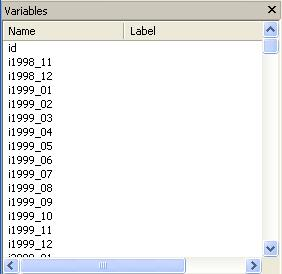You can export the data all together or by subsets. Let’s export in two files, one for returns and another for interest.

The following will export the return data into a *.csv file (readable in excel)

outsheet id r* using testr.csv, comma replace

Now the interest data

outsheet i* using testr.csv, comma replace

You can open these two files in excel.

Noticed the name of the files and beware of the path. The format above will save the files in the current active directory. To specify a path you will need to put it in quotation like

outsheet id r* using “H:\mydata\testr.csv”, comma replace

For more details on this command type

help reshape